# waynehu

## Professor, Department of Astronomy and Astrophysics University of Chicago

Group Contact CV SnapShots

## Basics

For pedagogical purposes, let us begin with an idealization of a perfect photon-baryon fluid and neglect the dynamical effects of gravity and the baryons. Perturbations in this perfect fluid can be described by a simple continuity and an Euler equation that encapsulate the basic properties of acoustic oscillations.

The discussion of acoustic oscillations will take place exclusively in Fourier space. For example, we decompose the monopole of the temperature field into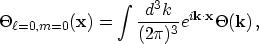(6)

and omit the subscripton the Fourier amplitude. Since perturbations are very small, the evolution equations are linear, and different Fourier modes evolve independently. Therefore, instead of partial differential equations for a field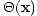, we have ordinary differential equations for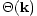. In fact, due to rotational symmetry, allfor a given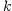obey the same equations. Here and in the following sections, we omit the wavenumber argumentwhere no confusion with physical space quantities will arise.

Temperature perturbations in Fourier space obey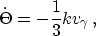(7)

This equation for the photon temperature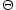, which does indeed look like the familiar continuity equation in Fourier space (derivatives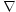become wavenumbers), has a number of subtleties hidden in it, due to the cosmological setting. First, the time'' derivative here is actually with respect to conformal time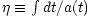. Since we are working in units in which the speed of light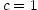,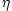is also the maximum comoving distance a particle could have traveled since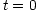. It is often called the comoving horizon or more specifically the comoving particle horizon. The physical horizon istimes the comoving horizon.

Second, the photon fluid velocity here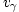has been written as a scalar instead of a vector. In the early universe, only the velocity component parallel to the wavevector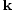is expected to be important, since they alone have a source in gravity. Specifically,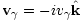. In terms of the moments introduced in §2,represents a dipole moment directed along. The factor of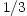comes about since continuity conserves photon number not temperature and the number density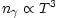. Finally, we emphasize that, for the time being, we are neglecting the effects of gravity.

The Euler equation for a fluid is an expression of momentum conservation. The momentum density of the photons is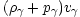, where the photon pressure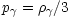. In the absence of gravity and viscous fluid imperfections, pressure gradients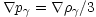supply the only force. Since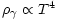, this becomes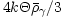in Fourier space. The Euler equation then becomes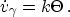(8)

Differentiating the continuity equation and inserting the Euler equation yields the most basic form of the oscillator equation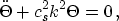(9)

where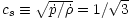is the sound speed in the (dynamically baryon-free) fluid. What this equation says is that pressure gradients act as a restoring force to any initial perturbation in the system which thereafter oscillate at the speed of sound. Physically these temperature oscillations represent the heating and cooling of a fluid that is compressed and rarefied by a standing sound or acoustic wave. This behavior continues until recombination. Assuming negligible initial velocity perturbations, we have a temperature distribution at recombination of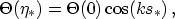(10)

where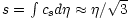is the distance sound can travel by, usually called the sound horizon. Asterisks denote evaluation at recombination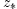.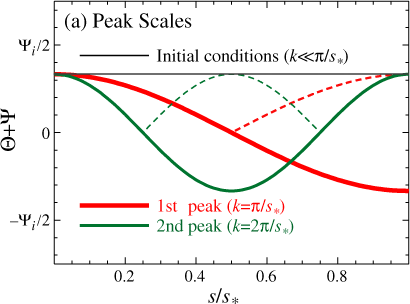Figure: Idealized acoustic oscillations. (a) Peak scales: the wavemode that completes half an oscillation by recombination sets the physical scale of the first peak. Both minima and maxima correspond to peaks in power (dashed lines, absolute value) and so higher peaks are integral multiples of this scale with equal height. Plotted here is the idealization of Equation (15) (constant potentials, no baryon loading).

In the limit of scales large compared with the sound horizon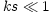, the perturbation is frozen into its initial conditions. This is the gist of the statement that the large-scale anisotropies measured by COBE directly measure the initial conditions. On small scales, the amplitude of the Fourier modes will exhibit temporal oscillations, as shown in Figure 1 [with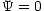,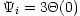for this idealization]. Modes that are caught at maxima or minima of their oscillation at recombination correspond to peaks in the power, i.e. the variance of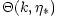. Because sound takes half as long to travel half as far, modes corresponding to peaks follow a harmonic relationship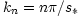, whereis an integer (see Figure 1a).

How does this spectrum of inhomogeneities at recombination appear to us today? Roughly speaking, a spatial inhomogeneity in the CMB temperature of wavelength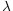appears as an angular anisotropy of scale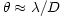where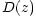is the comoving angular diameter distance from the observer to redshift. We will address this issue more formally in §3.8. In a flat universe,, where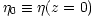. In harmonic space, the relationship implies a coherent series of acoustic peaks in the anisotropy spectrum, located at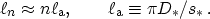(11)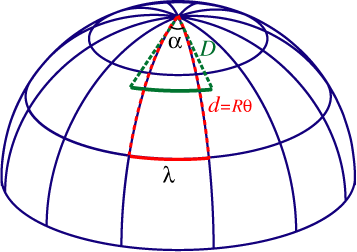Figure: Angular diameter distance. In a closed universe, objects are further than they appear to be from Euclidean (flat) expectations corresponding to the difference between coordinate distance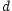and angular diameter distance. Consequently, at a fixed coordinate distance, a given angle corresponds to a smaller spatial scale in a closed universe. Acoustic peaks therefore appear at larger angles or lower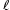in a closed universe. The converse is true for an open universe.

To get a feel for where these features should appear, note that in a flat matter dominated universe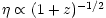so that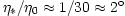. Equivalently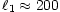. Notice that since we are measuring ratios of distances the absolute distance scale drops out; we shall see in §3.5 that the Hubble constant sneaks back into the problem because the Universe is not fully matter-dominated at recombination.

In a spatially curved universe, the angular diameter distance no longer equals the coordinate distance making the peak locations sensitive to the spatial curvature of the Universe [Doroshkevich et al, 1978,Kamionkowski et al, 1994]. Consider first a closed universe with radius of curvature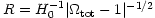. Suppressing one spatial coordinate yields a 2-sphere geometry with the observer situated at the pole (see Figure 2). Light travels on lines of longitude. A physical scaleat fixed latitude given by the polar angle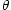subtends an angle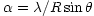. For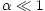, a Euclidean analysis would infer a distance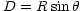, even though the coordinate distance along the arc is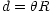; thus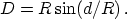(12)

For open universes, simply replace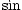with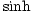. The result is that objects in an open (closed) universe are closer (further) than they appear, as if seen through a lens. In fact one way of viewing this effect is as the gravitational lensing due to the background density (c.f. §4.2.4). A given comoving scale at a fixed distance subtends a larger (smaller) angle in a closed (open) universe than a flat universe. This strong scaling with spatial curvature indicates that the observed first peak atconstrains the geometry to be nearly spatially flat. We will implicitly assume spatial flatness in the following sections unless otherwise stated.

Finally in a flat dark energy dominated universe, the conformal age of the Universe decreases approximately as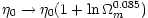. For reasonable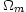, this causes only a small shift of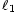to lower multipoles (see Plate 4) relative to the effect of curvature. Combined with the effect of the radiation near recombination, the peak locations provides a means to measure the physical age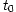of a flat universe [Hu et al, 2001].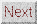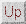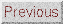Next: Initial Conditions Up: ACOUSTIC PEAKS Previous: ACOUSTIC PEAKS
Wayne Hu 2001-10-15